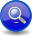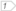#Boost C++ Libraries

...one of the most highly regarded and expertly designed C++ library projects in the world.

#### Warming up

We'll start by showing examples of parser expressions to give you a feel on how to build parsers from the simplest parser, building up as we go. When comparing EBNF to Spirit, the expressions may seem awkward at first. Spirit heavily uses operator overloading to accomplish its magic.

###### Trivial Example #1 Parsing a number

Create a parser that will parse a floating-point number.

```double_
```

(You've got to admit, that's trivial!) The above code actually generates a Spirit floating point parser (a built-in parser). Spirit has many pre-defined parsers and consistent naming conventions help you keep from going insane!

###### Trivial Example #2 Parsing two numbers

Create a parser that will accept a line consisting of two floating-point numbers.

```double_ >> double_
```

Here you see the familiar floating-point numeric parser `double_` used twice, once for each number. What's that `>>` operator doing in there? Well, they had to be separated by something, and this was chosen as the "followed by" sequence operator. The above program creates a parser from two simpler parsers, glueing them together with the sequence operator. The result is a parser that is a composition of smaller parsers. Whitespace between numbers can implicitly be consumed depending on how the parser is invoked (see below).

NoteWhen we combine parsers, we end up with a "bigger" parser, but it's still a parser. Parsers can get bigger and bigger, nesting more and more, but whenever you glue two parsers together, you end up with one bigger parser. This is an important concept.
###### Trivial Example #3 Parsing zero or more numbers

Create a parser that will accept zero or more floating-point numbers.

```*double_
```

This is like a regular-expression Kleene Star, though the syntax might look a bit odd for a C++ programmer not used to seeing the `*` operator overloaded like this. Actually, if you know regular expressions it may look odd too since the star is before the expression it modifies. C'est la vie. Blame it on the fact that we must work with the syntax rules of C++.

Any expression that evaluates to a parser may be used with the Kleene Star. Keep in mind that C++ operator precedence rules may require you to put expressions in parentheses for complex expressions. The Kleene Star is also known as a Kleene Closure, but we call it the Star in most places.

###### Trivial Example #4 Parsing a comma-delimited list of numbers

This example will create a parser that accepts a comma-delimited list of numbers.

```double_ >> *(char_(',') >> double_)
```

Notice `char_(',')`. It is a literal character parser that can recognize the comma `','`. In this case, the Kleene Star is modifying a more complex parser, namely, the one generated by the expression:

```(char_(',') >> double_)
```

Note that this is a case where the parentheses are necessary. The Kleene star encloses the complete expression above.

###### Let's Parse!

We're done with defining the parser. So the next step is now invoking this parser to do its work. There are a couple of ways to do this. For now, we will use the `phrase_parse` function. One overload of this function accepts four arguments:

1. An iterator pointing to the start of the input
2. An iterator pointing to one past the end of the input
3. The parser object
4. Another parser called the skip parser

In our example, we wish to skip spaces and tabs. Another parser named `space` is included in Spirit's repertoire of predefined parsers. It is a very simple parser that simply recognizes whitespace. We will use `space` as our skip parser. The skip parser is the one responsible for skipping characters in between parser elements such as the `double_` and `char_`.

Ok, so now let's parse!

```template <typename Iterator>
bool parse_numbers(Iterator first, Iterator last)
{
using qi::double_;
using qi::phrase_parse;
using ascii::space;

bool r = phrase_parse(
first,last,double_ >> *(',' >> double_),space);
if (first != last) // fail if we did not get a full match
return false;
return r;
}
```

 start iterator end iterator the parser the skip-parser

The parse function returns `true` or `false` depending on the result of the parse. The first iterator is passed by reference. On a successful parse, this iterator is repositioned to the rightmost position consumed by the parser. If this becomes equal to `last`, then we have a full match. If not, then we have a partial match. A partial match happens when the parser is only able to parse a portion of the input.

Note that we inlined the parser directly in the call to parse. Upon calling parse, the expression evaluates into a temporary, unnamed parser which is passed into the parse() function, used, and then destroyed.

Here, we opted to make the parser generic by making it a template, parameterized by the iterator type. By doing so, it can take in data coming from any STL conforming sequence as long as the iterators conform to a forward iterator.

You can find the full cpp file here: ../../example/qi/num_list1.cpp

Note`char` and `wchar_t` operands The careful reader may notice that the parser expression has `','` instead of `char_(',')` as the previous examples did. This is ok due to C++ syntax rules of conversion. There are `>>` operators that are overloaded to accept a `char` or `wchar_t` argument on its left or right (but not both). An operator may be overloaded if at least one of its parameters is a user-defined type. In this case, the `double_` is the 2nd argument to `operator>>`, and so the proper overload of `>>` is used, converting `','` into a character literal parser. The problem with omitting the `char_` should be obvious: ```'a' >> 'b'``` is not a spirit parser, it is a numeric expression, right-shifting the ASCII (or another encoding) value of `'a'` by the ASCII value of `'b'`. However, both ```char_('a') >> 'b'``` and ```'a' >> char_('b')``` are Spirit sequence parsers for the letter `'a'` followed by `'b'`. You'll get used to it, sooner or later.

Finally, take note that we test for a full match (i.e. the parser fully parsed the input) by checking if the first iterator, after parsing, is equal to the end iterator. You may strike out this part if partial matches are to be allowed.